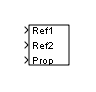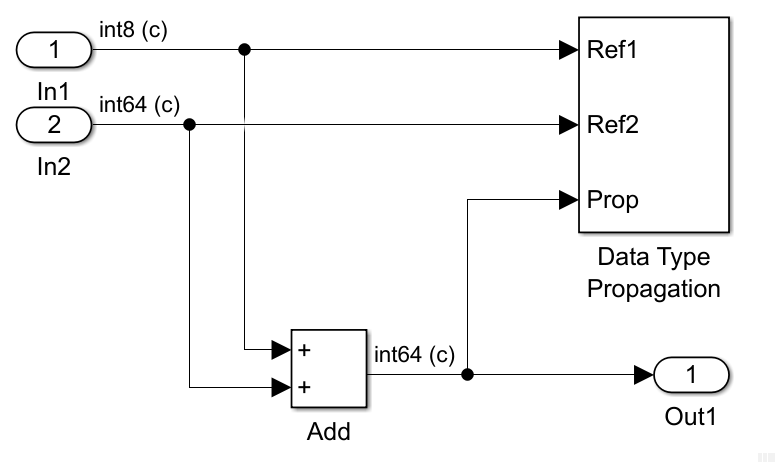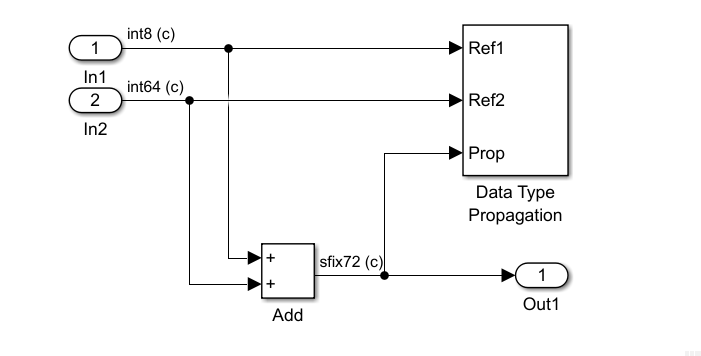# Data Type Propagation

Set data type and scaling of propagated signal based on information from reference signals

•Libraries:
HDL Coder / Signal Attributes

## Description

The Data Type Propagation block allows you to control the data type and scaling of signals in your model. You can use this block along with fixed-point blocks that have their Output data type parameter configured to `Inherit: Inherit via back propagation`.

The block has three inputs: Ref1 and Ref2 are the reference inputs, while the Prop input back-propagates the data type and scaling information gathered from the reference inputs. This information is then passed on to other fixed-point blocks.

The block provides many choices for propagating data type and scaling information. For example, you can use:

• The number of bits from the Ref1 reference signal or the number of bits from widest reference signal

• The range from the Ref2 reference signal or the range of the reference signal with the greatest range

• A bias of zero, regardless of the biases used by the reference signals

• The precision of the reference signal with the least precision

You specify how data type information is propagated using the Propagated data type parameter:

• If you select `Specify via dialog`, then you manually specify the data type via the Propagated data type edit field.

• If you select `Inherit via propagation rule`, then you must use the parameters described in Parameters.

You specify how scaling information is propagated using the Propagated scaling parameter:

• If you select `Specify via dialog`, then you manually specify the scaling via the Propagated scaling edit field.

• If you select `Inherit via propagation rule`, then you must use the parameters described in Parameters.

After you use the information from the reference signals, you can apply a second level of adjustments to the data type and scaling. To do so, use individual multiplicative and additive adjustments. This flexibility has various uses. For example, if you are targeting a DSP, then you can configure the block so that the number of bits associated with a multiply and accumulate (MAC) operation is twice as wide as the input signal, and has a specific number of guard bits added to it.

The Data Type Propagation block also provides a mechanism to force the computed number of bits to a useful value. For example, if you are targeting a 16-bit micro, then the target C compiler is likely to support sizes of only 8 bits, 16 bits, and 32 bits. The block forces these three choices to be used. For example, suppose that the block computes a data type size of 24 bits. Since 24 bits is not directly usable by the target chip, the signal is forced up to 32 bits, which is natively supported.

There is also a method for dealing with floating-point reference signals. This method makes it easier to create designs that are easily retargeted between fixed-point chips and floating-point chips.

The Data Type Propagation block allows you to set up libraries of useful subsystems that are properly configured based on the connected signals. Without this data type propagation process, subsystems from a library are unlikely to work as desired with most integer or fixed-point signals. Manual intervention would be required to configure the data type and scaling. In many situations, this block can eliminate the manual intervention.

### Precedence Rules

The precedence of the dialog box parameters decreases from top to bottom. Also:

• Double-precision reference inputs have precedence over all other data types.

• Single-precision reference inputs have precedence over integer and fixed-point data types.

• The number of bits is determined before the precision or positive range is inherited from the reference inputs.

• `PosRange` is one bit higher than the exact maximum positive range of the signal.

• The computed number-of-bits are promoted to the smallest allowable value that is greater than or equal to the computation. If none exists, then the block returns an error.

## Examples

expand all

This example shows how to propagate data types in a Simulink model, using a Data Type Propagation block.

This model adds two numbers of datatypes `int8` and `int64`. The blocks in the model use the following parameters:

• In the block parameters for the Inport blocks, under the Signal Attributes tab, the first Inport's Data type is `int8` and the second Inport's Data type is `int64`.

• In the block parameters of the Add block, the Output data type is `Inherit: Inherit via back propagation`. This allows the Data Type Propagation block to define the output data type of the block.

• In the block parameters of the Data Type Propagation block, in the Propagated type tab, Propagated data type is `Inherit via propagation rule`.

• The data types of the inports are displayed by selecting Debug > Diagnostics > Information Overlays > PORTS > Base Data Types. This is used to show how the sum's data type changes under different parameters.

```open_system('DataTypePropagation') ```To observe how the Data Type Propagation block impacts the data type of the sum, modify the Block Parameters:

In the Block Parameters of the Data Type Propagation block, Number-of-Bits is set to `max([NumBits1 NumBits2])`. Press Ctrl-D to compile and update the model. Observe that the data type of the output is now `int64`, the max bits out of 8 bits and 64 bits.In the Block Parameters of the Data Type Propagation block, Number-of-Bits is set to `NumBits1+NumBits2`. Update the diagram again. Observe that the size of the output data type is now 72 bits, which is the sum of the input integer lengths.In the Block Parameters of the Data Type Propagation block, Is-Signed is set to `FALSE`. Update the diagram again. Observe that the data type of the output is now `ufix72`, an unsigned 72 bit integer.## Ports

### Input

expand all

First reference signal, from which to gather data type and scaling information.

Data Types: `single` | `double` | `int8` | `int16` | `int32` | `int64` | `uint8` | `uint16` | `uint32` | `uint64` | `Boolean` | `fixed point`

Second reference signal from which to gather data type and scaling information.

Data Types: `single` | `double` | `int8` | `int16` | `int32` | `int64` | `uint8` | `uint16` | `uint32` | `uint64` | `Boolean` | `fixed point`

Data type and scaling information, back-propagated to the model. After the block gathers data type and scaling information from the reference signals, you can apply a second level of adjustments to the data type and scaling. To do so, specify individual multiplicative and additive adjustments in the block dialog box.

Data Types: `single` | `double` | `int8` | `int16` | `int32` | `int64` | `uint8` | `uint16` | `uint32` | `uint64` | `fixed point`

## Parameters

expand all

### Propagated Type

Specify whether to propagate the data type via the dialog box, or inherit the data type from the reference signals.

#### Dependencies

Setting this parameter to ```Specify via dialog``` enables the 1.1. Propagated data type (e.g. fixdt(1,16), fixdt('single')).

#### Programmatic Use

 Block Parameter: `PropDataTypeMode` Type: character vector Values: ```'Specify via dialog' | 'Inherit via propagation rule'``` Default: `'Inherit via propagation rule'`

Specify the data type to propagate.

#### Dependencies

To enable this parameter, set 1. Propagated data type to ```Specify via dialog```.

#### Programmatic Use

 Block Parameter: `PropDataTypeMode` Type: character vector Values: ```'Specify via dialog' | 'Inherit via propagation rule'``` Default: `'Inherit via propagation rule'`

Specify the output data type as `single` or `double`. This parameter makes it easier to create designs that are easily retargeted between fixed-point chips and floating-point chips.

#### Dependencies

To enable this parameter, set Propagated data type to ```Inherit via propagation rule```.

#### Programmatic Use

 Block Parameter: `IfRefDouble` Type: character vector Values: `'double' | 'single'` Default: `'double'`

Specify the output data type as `single` or `double`. This parameter makes it easier to create designs that are easily retargeted between fixed-point chips and floating-point chips.

#### Dependencies

To enable this parameter, set Propagated data type to ```Inherit via propagation rule```.

#### Programmatic Use

 Block Parameter: `IfRefSingle` Type: character vector Values: `'double' | 'single'` Default: `'single'`

Specify the sign of Prop as one of the following values.

Parameter ValueDescription

`IsSigned1`

Prop is a signed data type if Ref1 is a signed data type.

`IsSigned2`

Prop is a signed data type if Ref2 is a signed data type.

```IsSigned1 or IsSigned2```

Prop is a signed data type if either Ref1 or Ref2 are signed data types.

`TRUE`

Ref1 and Ref2 are ignored, and Prop is always a signed data type.

`FALSE`

Ref1 and Ref2 are ignored, and Prop is always an unsigned data type.

For example, if the Ref1 signal is `ufix(16)`, the Ref2 signal is `sfix(16)`, and the Is-Signed parameter is `IsSigned1 or IsSigned2`, then Prop is forced to be a signed data type.

#### Dependencies

To enable this parameter, set Propagated data type to ```Inherit via propagation rule```.

#### Programmatic Use

 Block Parameter: `IsSigned` Type: character vector Values: ```'IsSigned1' | 'IsSigned2' | 'IsSigned1 or IsSigned2' | 'TRUE' | 'FALSE'``` Default: `'IsSigned1 or IsSigned2'`

Specify the number of bits used by Prop for the base data type as one of the following values.

Parameter ValueDescription

`NumBits1`

The number of bits for Prop is given by the number of bits for Ref1.

`NumBits2`

The number of bits for Prop is given by the number of bits for Ref2.

```max([NumBits1 NumBits2])```

The number of bits for Prop is given by the reference signal with largest number of bits.

```min([NumBits1 NumBits2])```

The number of bits for Prop is given by the reference signal with smallest number of bits.

`NumBits1+NumBits2`

The number of bits for Prop is given by the sum of the reference signal bits.

#### Dependencies

To enable this parameter, set Propagated data type to ```Inherit via propagation rule```.

#### Programmatic Use

 Block Parameter: `NumBitsBase` Type: character vector Values: ```'NumBits1' | 'NumBits2' | 'max([NumBits1 NumBits2])' | 'min([NumBits1 NumBits2])' | 'NumBits1+NumBits2'``` Default: `'max([NumBits1 NumBits2])'`

Specify the number of bits used by Prop by including a multiplicative adjustment that uses a data type of `double`. For example, suppose that you want to guarantee that the number of bits associated with a multiply and accumulate (MAC) operation is twice as wide as the input signal. To do this, set this parameter to `2`.

#### Dependencies

To enable this parameter, set Propagated data type to ```Inherit via propagation rule```.

#### Programmatic Use

 Block Parameter: `NumBitsMult` Type: character vector Values: positive integer Default: `'1'`

Specify the number of bits used by Prop by including an additive adjustment that uses a data type of `double`. For example, if you are performing multiple additions during a MAC operation, the result could overflow. To prevent overflow, you can associate guard bits with the propagated data type. To associate four guard bits, you specify the value `4`.

#### Dependencies

To enable this parameter, set Propagated data type to ```Inherit via propagation rule```.

#### Programmatic Use

 Block Parameter: `NumBitsAdd` Type: character vector Values: scalar Default: `'0'`

Force the computed number of bits used by Prop to a useful value. For example, if you are targeting a processor that supports only 8 bits, 16 bits, and 32 bits, then you configure this parameter to `[8,16,32]`. The block always propagates the smallest specified value that fits. If you want to allow all fixed-point data types, you would specify the value `1:128`.

#### Dependencies

To enable this parameter, set Propagated data type to ```Inherit via propagation rule```.

#### Programmatic Use

 Block Parameter: `NumBitsAllowFinal` Type: character vector Values: scalar or vector of positive integers Default: `'1:128'`

### Propagated Scaling

Choose to propagate the scaling via the dialog box, inherit the scaling from the reference signals, or calculate the scaling to obtain best precision.

#### Programmatic Use

 Block Parameter: `PropScalingMode` Type: character vector Values: `Inherit via propagation rule` | `Specify via dialog` | ```Obtain via best precision``` Default: `Inherit via propagation rule`

Specify the scaling as either a slope or a slope and bias.

#### Dependencies

To enable this parameter, set Propagated scaling to ```Specify via dialog```.

#### Programmatic Use

 Block Parameter: `PropScaling` Type: character vector Values: `Slope` | ```[Slope Bias]``` Default: `'2^-10'`

Specify any values to be used to constrain the precision, such as the upper and lower limits on the propagated input. Based on the data type, the block selects a scaling such that these values can be represented with no overflow error and minimum quantization error.

#### Dependencies

To enable this parameter, set Propagated scaling to ```Obtain via best precision```.

#### Programmatic Use

 Block Parameter: `ValuesUsedBestPrec` Type: character vector Values: vector of values Default: `'[5 -7]'`

Specify the slope used by Prop for the base data type as one of the following values.

Parameter ValueDescription

`Slope1`

The slope of Prop is given by the slope of Ref1.

`Slope2`

The slope of Prop is given by the slope of Ref2.

```max([Slope1 Slope2])```

The slope of Prop is given by the maximum slope of the reference signals.

```min([Slope1 Slope2])```

The slope of Prop is given by the minimum slope of the reference signals.

`Slope1*Slope2`

The slope of Prop is given by the product of the reference signal slopes.

`Slope1/Slope2`

The slope of Prop is given by the ratio of the Ref1 slope to the Ref2 slope.

`PosRange1`

The range of Prop is given by the range of Ref1.

`PosRange2`

The range of Prop is given by the range of Ref2.

```max([PosRange1 PosRange2])```

The range of Prop is given by the maximum range of the reference signals.

```min([PosRange1 PosRange2])```

The range of Prop is given by the minimum range of the reference signals.

`PosRange1*PosRange2`

The range of Prop is given by the product of the reference signal ranges.

`PosRange1/PosRange2`

The range of Prop is given by the ratio of the Ref1 range to the Ref2 range.

You control the precision of Prop with `Slope1` and `Slope2`, and you control the range of Prop with `PosRange1` and `PosRange2`. Also, `PosRange1` and `PosRange2` are one bit higher than the maximum positive range of the associated reference signal.

#### Dependencies

To enable this parameter, set Propagated scaling to ```Inherit via propagation rule```.

#### Programmatic Use

 Block Parameter: `SlopeBase` Type: character vector Values: ```'Slope1' | 'Slope2' | 'max([Slope1 Slope2])' | 'min([Slope1 Slope2])' | 'Slope1*Slope2' | 'Slope1/Slope2' | 'PosRange1' | 'PosRange2' | 'max([PosRange1 PosRange2])' | 'min([PosRange1 PosRange2])' | 'PosRange1*PosRange2' | 'PosRange1/PosRange2'``` Default: `'min([Slope1 Slope2])'`

Specify the slope used by Prop by including a multiplicative adjustment that uses a data type of `double`. For example, if you want 3 bits of additional precision (with a corresponding decrease in range), the multiplicative adjustment is `2^-3`.

#### Dependencies

To enable this parameter, set Propagated scaling to ```Inherit via propagation rule```.

#### Programmatic Use

 Block Parameter: `SlopeMult` Type: character vector Values: scalar Default: `'1'`

Specify the slope used by Prop by including an additive adjustment that uses a data type of `double`. An additive slope adjustment is often not needed. The most likely use is to set the multiplicative adjustment to `0`, and set the additive adjustment to force the final slope to a specified value.

#### Dependencies

To enable this parameter, set Propagated scaling to ```Inherit via propagation rule```.

#### Programmatic Use

 Block Parameter: `SlopeAdd` Type: character vector Values: scalar Default: `'0'`

Specify the bias used by Prop for the base data type. The parameter values are described as follows:

Parameter ValueDescription

`Bias1`

The bias of Prop is given by the bias of Ref1.

`Bias2`

The bias of Prop is given by the bias of Ref2.

```max([Bias1 Bias2])```

The bias of Prop is given by the maximum bias of the reference signals.

```min([Bias1 Bias2])```

The bias of Prop is given by the minimum bias of the reference signals.

`Bias1*Bias2`

The bias of Prop is given by the product of the reference signal biases.

`Bias1/Bias2`

The bias of Prop is given by the ratio of the Ref1 bias to the Ref2 bias.

`Bias1+Bias2`

The bias of Prop is given by the sum of the reference biases.

`Bias1-Bias2`

The bias of Prop is given by the difference of the reference biases.

#### Dependencies

To enable this parameter, set Propagated scaling to ```Inherit via propagation rule```.

#### Programmatic Use

 Block Parameter: `BiasBase` Type: character vector Values: ```'Bias1' | 'Bias2' | 'max([Bias1 Bias2])' | 'min([Bias1 Bias2])' | 'Bias1*Bias2' | 'Bias1/Bias2' | 'Bias1+Bias2' | 'Bias1-Bias2'``` Default: `'Bias1'`

Specify the bias used by Prop by including a multiplicative adjustment that uses a data type of `double`.

This parameter is visible only when you set Propagated scaling to ```Inherit via propagation rule```.

#### Programmatic Use

 Block Parameter: `BiasMult` Type: character vector Values: scalar Default: `'1'`

Specify the bias used by Prop by including an additive adjustment that uses a data type of `double`.

If you want to guarantee that the bias associated with Prop is zero, configure both the multiplicative adjustment and the additive adjustment to `0`.

#### Dependencies

To enable this parameter, set Propagated scaling to ```Inherit via propagation rule```.

#### Programmatic Use

 Block Parameter: `BiasAdd` Type: character vector Values: scalar Default: `'0'`

## Block Characteristics

 Data Types `Boolean` | `double` | `fixed point` | `half` | `integer` | `single` Direct Feedthrough `no` Multidimensional Signals `yes` Variable-Size Signals `yes` Zero-Crossing Detection `no`

## Version History

Introduced before R2006a